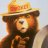# Convert Zero Lag MACD from TradingView

L

#### Likos

##### Member
2019 Donor
By far, my favorite substudy. I would love to use this on TOS but I've no idea how to convert the script to TOS. Here are the details below.

Rich (BB code):
``````// ENHANCED ZERO LAG MACD
// Version 1.1
//
// Based on ZeroLag EMA - see Technical Analysis of Stocks and Commodities, April 2000
// Original version by user Glaz. Thanks ! https://www.tradingview.com/chart/EURUSD/UV0YI6Wy-ZeroLag-Macd
// Ideas and code from @yassotreyo version.
// Tweaked by Albert Callisto (AC)
//
// Last Update 20.11.2016
// (AC - 1.0) Histogram with two colors, choice between SMA/EMA (SMA = "Glaz mode"),  names for sub-components, renaming of variables
// (AC - 1.1) Added choice between "Glaz" and legacy algorithm + introduced EMA on MACD (thanks @yassotreyo for your original version)

study(title="Zero Lag MACD Enhanced - Version 1.1", shorttitle="Zero Lag MACD Enhanced 1.1")
source = close

fastLength = input(12, minval=1)
slowLength = input(26, minval=1)
signalLength =input(9, minval=1)
MacdEmaLength =input(9, minval=1)
useEma = input(true, title="Use EMA (otherwise SMA)")
useOldAlgo = input(false, title="Use Glaz algo (otherwise 'real' original zero lag)")

// Fast line
ma1= useEma ? ema(source, fastLength) : sma(source, fastLength)
ma2 = useEma ?  ema(ma1,fastLength) :  sma(ma1,fastLength)
zerolagEMA = ((2 * ma1) - ma2)

// Slow line
mas1=  useEma ? ema(source , slowLength) :  sma(source , slowLength)
mas2 =  useEma ? ema(mas1 , slowLength): sma(mas1 , slowLength)
zerolagslowMA = ((2 * mas1) - mas2)

// MACD line
ZeroLagMACD = zerolagEMA - zerolagslowMA

// Signal line
emasig1 = ema(ZeroLagMACD, signalLength)
emasig2 = ema(emasig1, signalLength)
signal = useOldAlgo ? sma(ZeroLagMACD, signalLength) : (2 * emasig1) - emasig2

hist = ZeroLagMACD - signal

upHist = (hist > 0) ? hist : 0
downHist = (hist <= 0) ? hist : 0

p1 = plot(upHist, color=green, transp=40, style=columns, title='Positive delta')
p2 = plot(downHist, color=purple, transp=40, style=columns, title='Negative delta')

zeroLine = plot(ZeroLagMACD, color=black, transp=0, linewidth=2, title='MACD line')
signaLine = plot(signal, color=gray, transp=0, linewidth=2, title='Signal')

ribbonDiff = hist > 0 ? green : purple
fill(zeroLine, signaLine, color=ribbonDiff)

plot(ema(ZeroLagMACD,MacdEmaLength) , color=red, transp=0, linewidth=2, title='EMA on MACD line')``````

Last edited by a moderator:#### BenTen

Staff
VIP
There is also an existing Zero Lag MACD indicator for ThinkorSwim. Here you go.

Rich (BB code):
``````# Super Zero Lag MACD
# Mobius

declare lower;
input Fast_Length = 5;
input Slow_Length = 13;
input MACD_Length = 3;
plot MACD5 = (MACD("fast length" = Fast_Length,
"slow length" = Slow_Length,
"macd length" = MACD_Length).Avg /
StDev(MACD("fast length" = Fast_Length,
"slow length" = Slow_Length,
"macd length" = MACD_Length).Diff, Slow_Length));
MACD5.SetDefaultColor(Color.CYAN);
plot Squeeze = if BollingerBandsSMA().UpperBand < KeltnerChannels().Upper_Band
then 0
else Double.NaN;
Squeeze.SetPaintingStrategy(PaintingStrategy.POINTS);
Squeeze.SetLineWeight(3);
Squeeze.SetDefaultColor(Color.GREEN);
plot zero = if IsNaN(close) or !IsNaN(Squeeze) then Double.NaN else 0;
zero.SetPaintingStrategy(PaintingStrategy.POINTS);
zero.SetLineWeight(2);
zero.SetDefaultColor(Color.BLUE);
AddLabel(!IsNaN(Squeeze), "Squeeze", if MACD5 > MACD5 and
MACD5 < 0
then Color.GREEN
else Color.RED);
def day = GetValue(GetYYYYMMDD(), 1);
def trend = if close > (Highest(high, 21) + Lowest(low, 21)) / 2
then 1
else 0;
then "Trend Up"
else "Trend Dn",
if trend == 1
then Color.Green
else Color.Red);``````

Last edited:
•Hustle and markos
L

#### Likos

##### Member
2019 Donor
@BenTen I love you

Last edited:
•markos
L

#### Likos

##### Member
2019 Donor
@BenTen But personally, I like the tradingview one better because the orbs can share when it reaches its maximum potential, buying trend, or etc. The Mobius one I'm not too sure how to use this quite yet.

Last edited:How do I convert this ATR% chart study into a scanner watchlist column? Questions 5